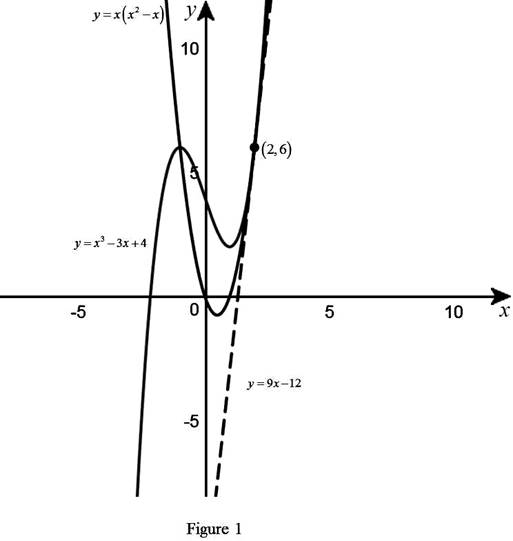# The point of the tangent line and sketch the graph of the curve and tangent line.### Single Variable Calculus: Concepts...

4th Edition
James Stewart
Publisher: Cengage Learning
ISBN: 9781337687805### Single Variable Calculus: Concepts...

4th Edition
James Stewart
Publisher: Cengage Learning
ISBN: 9781337687805

#### Solutions

Chapter 3, Problem 2P
To determine

## To find: The point of the tangent line and sketch the graph of the curve and tangent line.

Expert Solution

The equation of the tangent line to the curve at (2,6) is, y=9x12.### Explanation of Solution

Given:

The curves is y=x33x+4 and y=3(x2x).

Formula used:

The equation of the tangent line at (x1,y1) is, yy1=m(xx1) (1)

Where, m is the slope of the tangent line at (x1,y1) and m=dydx|x=x1,y=y1

Calculation:

Obtain the derivative of y1=x33x+4.

dy1dx=ddx(x33x+4)=3x313x11+0=3x23

Obtain the derivative of y2=3(x2x).

dy2dx=ddx(3(x2x))=3(2x211x11)=6x3

Since the slopes of the tangent of the two curves are equal. That is dy1dx=dy2dx.

3x23=6x33x26x=03x(x2)=0x=0 or x=2

Thus, the derivative of the equation is dy1dx=3x23 and dy2dx=6x3.

The slope of tangent to the curves at x=0 in dy1dx=3x23 .

dy1dx=3(0)23=03=3

The slope of the tangent is −3 but curve do not intersect.

The slope of tangent to the curves at x=2 in dy2dx=6x3.

dy1dx=6(2)3=123=9

The slope of the tangent is m=9 and the curve intersect at point (2,6).

Thus, the common tangent line at (2,6).

Substitute (2,6) for (x1,y1) and slope m=9 in equation (1),

y6=9(x2)y6=9x18y=9x18+6y=9x12

Thus, the equation of the tangent line to the curve at (2,6) is y=9x12.

Therefore, the required point is, (2,6).

Use the online graphing calculator and draw the given curves and obtained tangent line as shown in Figure 1.From Figure 1, it is observed that the given curves y=x33x+4 and y=3(x2x) are tangent to each other at the point (2,6).

### Have a homework question?

Subscribe to bartleby learn! Ask subject matter experts 30 homework questions each month. Plus, you’ll have access to millions of step-by-step textbook answers!